# [Interactive] How Hard Could Rocket Science Be, Anyway? | Orbital Mechanics in Roblox 1

Hey folks! It’s been a while since I made my previous unnecessarily complex engineering project here on the Blox. For my next project, I wanted to ask a simple question…

# How Hard Could Rocket Science Really Be, Anyway? | Orbital Mechanics in Roblox 1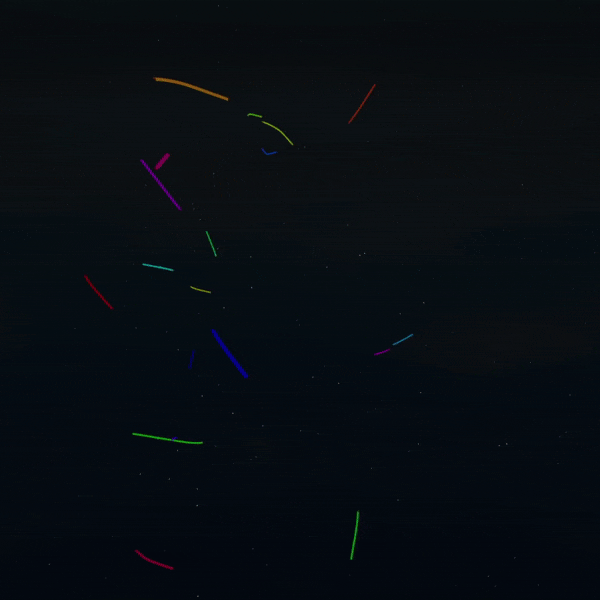## The Goal

The goal of this project is to build a game where the player designs and flies spacecraft under the laws of physics as they exist in the real world. If you have ever played games like Kerbal Space Program or SimpleRockets 2, you’ll have a general idea for what this project aims to achieve. If not, all you need to know is that this game is going to model real rocket science with code! I’m excited to share this journey with you, and I hope you pick up some knowledge about space, programming, and aerospace engineering along the way.

Before we make an orbital mechanics game, we need code that can simulate orbital mechanics. This devblog series will discuss the engineering and techniques behind making an orbital mechanics solver in Roblox.

BUT, WHY ROBLOX...?

Roblox obviously isn’t the greatest platform for making something like this. However, I chose Roblox anyway because I find great joy and excitement in making Roblox do things it was never designed to do. Working in a computationally-constrained environment like Roblox Lua makes creative problem solving and good engineering a necessity. At the end of the day, these out-of-the-box solutions are what makes me improve my skills and become a better engineer.

Enough introduction, let’s get into it!

## Orbital Mechanics Crash Course

Orbital Mechanics, also called Astrodynamics, is a subfield of physics that deals with modelling and predicting the motion of planets, spacecraft, comets, and other objects that fly through space.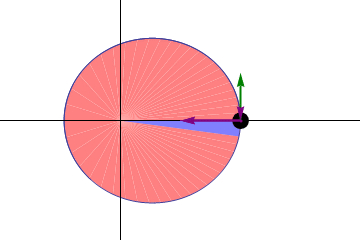A VERY BRIEF HISTORY OF ORBITAL MECHANICS

Humans have looked to the skies and have studied the movements of planets since the Neolithic Era. Understanding astronomy was critical to timekeeping, agriculture, navigation, spiritual worship, and more. Over the ages, a model for the solar system began to form. At first, it was common belief that the Earth was the center of the universe, and all heavenly bodies orbited it in perfectly circular orbits.

As an aside: computing and astronomy saw an unlikely intersection during antiquity. The Antikythera Mechanism, discovered in Greece, is the earliest known example of an analog mechanical computer. Its purpose was to predict the motion of planets in the sky, and modern replicas of the device confirm that it could produce accurate results over decades in advance.

Thousands of years would pass until Copernicus proposed the Copernican Model. Copernicus placed the sun at the center of the solar system, with all planets following circular orbits around it.

Our modern understanding of orbital mechanics began to coalesce in the late 1500’s, when the German Scientist Johannes Kepler derived his Laws of Planetary Motion from the years of astronomical observations collected by Tycho Brahe. Rather than circular, Kepler described orbits as elliptical, with the sun at a focus. He also came up with relations for orbital period and distance travelled over time.

Kepler’s Laws provided a sound description of orbits, but they did not offer a driving mechanism for the motion. In 1687, Newton discovered and described the missing context to these laws: Newtonian Mechanics, and the Universal Law of Gravitation. That brings us to the next section!

###INTERACTIVE: Newton and The Universal Law of GravitationGravity is one of the fundamental forces of the universe, and it is the force that drives motion within orbits. Everything in the universe with mass exerts a gravitational force on everything else with mass. The individual atoms in your body even exert gravitational force on one another, however small.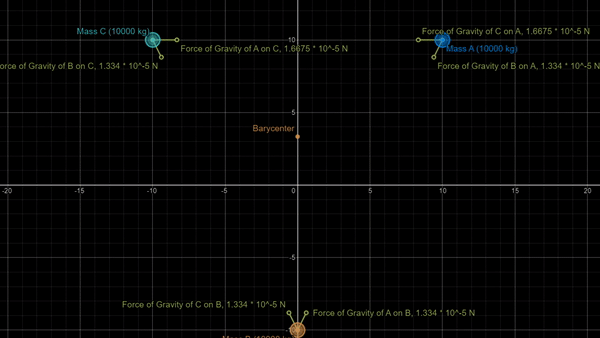Every gravitational interaction is equal and opposite. This means that you exert as much gravitational force on the Earth as the Earth exerts on you! However, the Earth doesn’t move towards you because it is much more massive. You can experiment with this idea in the demo by making one of the masses big, one of the masses small, and then enabling the acceleration visualization.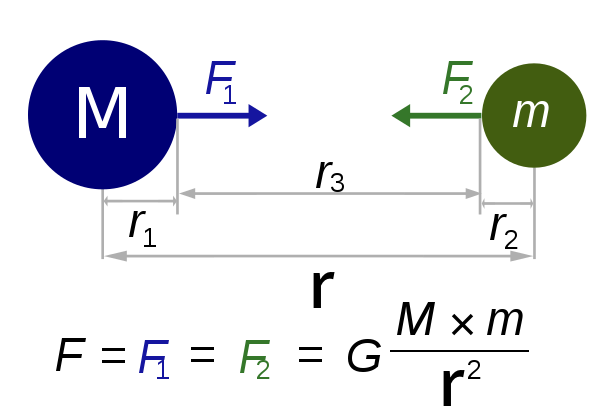Gravity is an inverse square law. This means that as the distance between two objects doubles, their gravitational force will decrease to 25% of its original strength. In the demo, I invite you to move one of the mass really far from the others to experience how quickly this happens. Also try moving the masses together. What happens?

##INTERACTIVE: The Chaotic Choreography of Reality: The N-Body UniverseAs we discussed with Newton’s Law of Universal Gravitation, all matter is subject to the force of gravity. In a galaxy, there might be billions of stars all pulling one another closer together. If we sat and watched these stars for billions of years pulling each other closer, what might we see?

Enter: the n-body gravitation simulation. The ‘n’ just means “an arbitrary number of objects.”

Using an iterative approach to roughly simulate an n-body system of gravitational bodies happens to be fairly trivial, and, to demonstrate, I made an open source demo less than an hour! Click the above link to check it out! You can also check out the source code here if you’re curious about how it is put together.In the universe, disorder, or entropy, is the norm. However, given enough time and chance, objects can fall into rhythm. In this cosmic dance, this stability is strangely beautiful.

These objects managed to land in a stable orbit of one another: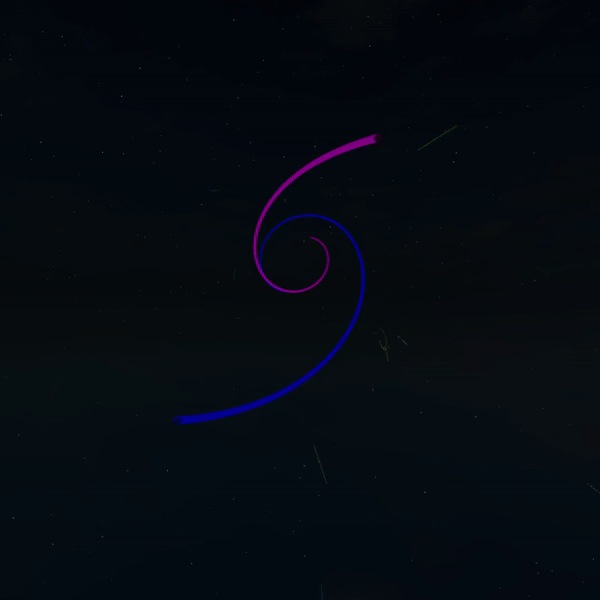These objects achieved a trinary orbital configuration, with two orbiting closely and one orbiting both on the periphery. Believe it or not, this happens in nature all the time; our closest interstellar neighbor, Alpha Centauri, follows a similar trinary orbital configuration: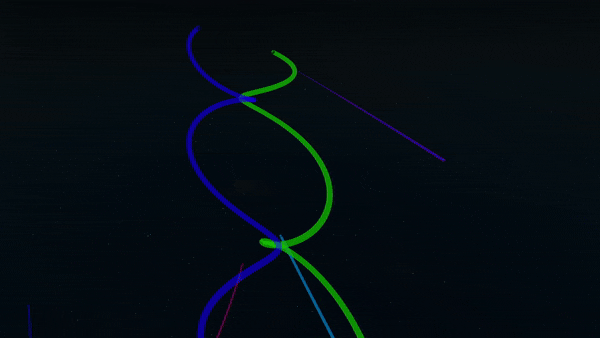### The Trouble with the N-Body Problem

Despite how simple it is to implement an n-body simulation iteratively, things start to get much more complex if we want to do any sort of analytical modelling. The problem with our iterative simulation is that each step of the simulation requires the previous step to be calculated beforehand. In other words, if we want to calculate the position of a planet after 450 steps (which is about 15 seconds of game time), we must calculate the previous 449 steps first.

This makes it impossible to do any sort of prediction (“After some time t, what is the object’s position?”) or optimization (“What is the closest two objects get to one another over the first 6 seconds?”). Moreover, time acceleration in an interative simulation is problematic. Either we can increase the amount of time that elapses between each step, (which leads to large degrees of inaccuracy over time) or we can solve more steps in the same amount of time (which is very computationally expensive.)

If we want to make a game about flying spacecraft, being unable to predict movement in the future is, to put it lightly, kind of an issue!

What we’re looking for is a mathematical equation that we can use to model the motion of an object at any given time with a single independent calculation. Turns out, general solutions for the n-body problem have been discovered, however their complexity is comparable to the chaos we saw in our simple simulation. NASA uses supercomputers to solve these equations. In Roblox, we obviously don’t have that type of hardware, so we’ll need to find some way to simplify the simulation.

##INTERACTIVE: The Two-Body ApproximationIn the gravity demo, we saw that the force of gravity falls off quickly with distance. If you watched the n-body demo, you might have notices that some objects fly off into the distance and only contribute a negligible amount of gravity to the rest of the objects in the universe. What if we ignored these mostly irrelevant bodies to reduce the complexity of our simulation?

Enter: the Two-Body Approximation!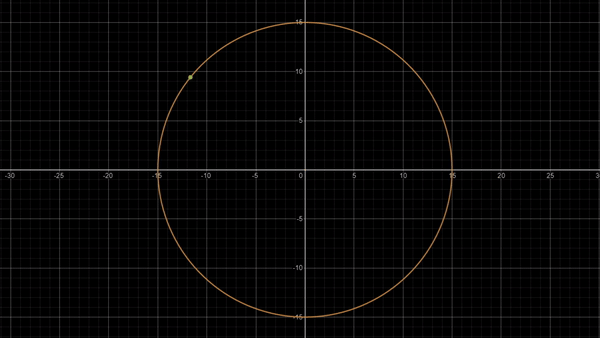In a two body system, we ignore all sources of gravity and objects except for two: the “parent body,” and the object orbiting it. The parent body can be anything of significant mass: a star, planet, moon, or even comet. The orbiting object can also be anything, but it should be something with significantly less mass than the parent body. This simplification logically follows what we normally think of when we think of orbits: one small object circling around a much bigger one.

EXAMPLES OF TWO-BODY SYSTEMS

Ignoring all sources of gravity beyond the two bodies in our system tremendously simplifies our calculations. If we start using Newton’s Universal Law of Gravitation, and then use vector math to solve it in terms of an angle, we get the Trajectory Equation: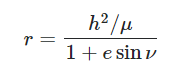…where ‘h’ is the specific angular momentum of the orbit, μ is the gravitational parameter (Gravitational Constant * Mass of Planet), and ‘e’ is eccentricity. The expression h²/μ also happens to be equivalent to the semilatus rectum, which we often denote as ‘p.’ The other variable, v, is called the “true anomaly,” and its an angle measure starting from the lowest part of the orbit going counterclockwise.

This expression is a polar conic equation. This means that trajectories in two-body systems happen to nicely follow the paths of the four conic sections. As conic sections are well-understood geometric forms, we will find that much of the math we will need to do in the future will be much easier.

Characterizing orbits in two dimensions are two important parameters:

• Eccentricity (e) is a measure of how deformed the orbit is from a circular shape. At e=0, the orbit is a perfect circle. When 0<e<1, the orbit is elliptical, with values closer to 1 leading to a more stretched shape. When e=1, the trajectory is actually an “escape trajectory,” meaning the object has so much speed that its distance from the parent will increase faster than gravity can pull the object back. And, when e>1, we get a hyperbolic trajectory.
• Semimajor Axis (a) is equivalent to half the length of an ellipse’s longest diameter. If eccentricity defines the shape of the orbit or trajectory, then the semimajor axis defines the actual size.

The values of these parameters can be calculated from initial velocity and position state vectors, but I’m going to avoid talking about the fine details behind the math. If you’re curious, feel free to ask in the comments!

## Spin Me Right Round: Orbits in Three Dimensions

So, we have a path for our orbit, but it only exists in two dimensions. But, orbits move in three dimensions.

An important insight here is that all the positions along a two-body orbits are coplanar. This means that every orbit can be represented as an orbit on a two-dimensional plane that is rotated in three-dimensional space.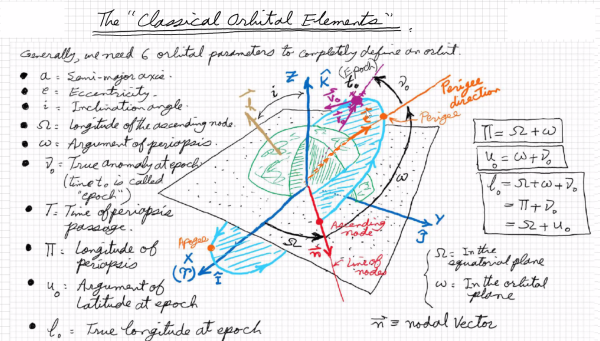To do this, we will introduce three more orbital parameters: Inclination (i), Longitude of the Ascending Node (Ω), and the Argument of the Periapsis (ω). Turns out, these three parameters have a one-to-one correspondence to a series of Euler Angle rotations. Euler Angles represent complex rotations as a sequence of single-axis rotations. The following diagram illustrates the idea pretty well; in the diagram’s case, we are applying a 45 degree rotation to the Z axis, then a 45 degree rotation to the Y axis, then finally a 45 degree rotation to the X axis.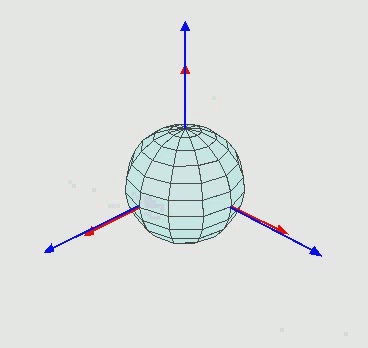In Roblox, how we typically apply rotations to parts is through the use of CFrames. However, at their core, CFrames are three-dimensional transformation matrices. We can speed things up by defining the matrix ourselves once, then applying the transformation to each point on our orbit curve.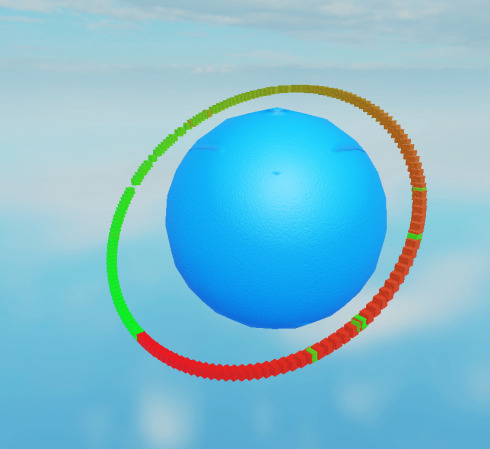The results speak for themselves; through simple rotation, we can now render any two-body orbit or escape trajectory.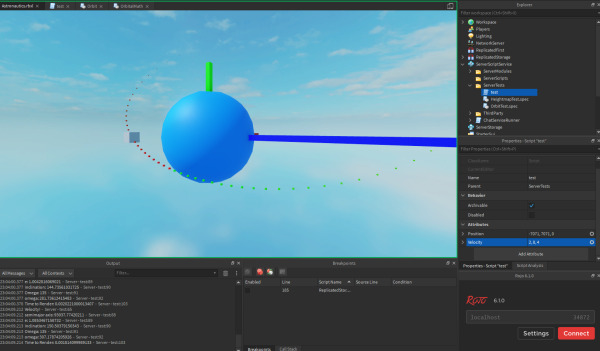Shout out to the crude 3-axis visualizer made out of parts. I needed that to visualize how to conduct the transformation.

## Next Time: Position as a Function of Time

Right now, we have a basic understanding of orbital mechanics, as well as a way to represent orbits as curves in Roblox. However, we still haven’t come up with a way to solve for an object’s position as a function of time in a two-body system. Turns out, that isn’t as straightforward as one might think. In the next blog, we will talk about the challenges of orbital prediction, along with some analytical solutions.

Until then, here’s a sneak peek: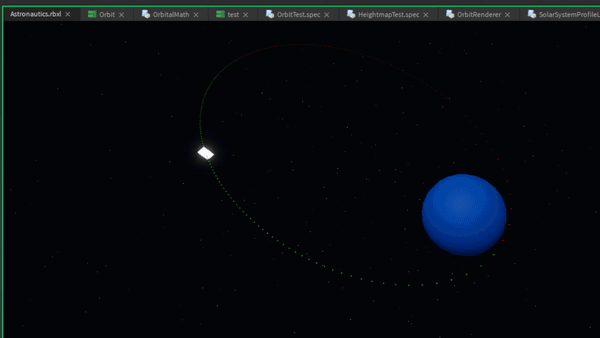Thanks for reading, and I hope to catch you again in the next installment!

## Acknowledgements

My good friend, Math major, and official Project Ourbits Mathematician @DarkInfernoDrago has been invaluable in teaching me about the mathematics necessary to implement this. He also straight-up derived several core parts of the solver. Without him, this project would not be possible!

Shout out to @Ukendio for coming up with the working title for this project. We probably won’t use it for the name of the game proper but it already is ubiquitous in our internal development files.

Shout out to everyone in the Computer Science Helpdesk channel for following the development of this project and being a sounding board for ideas.

68 Likes

Should this be in #resources:community-tutorials ?
btw cool

Should this be in #resources:community-tutorials ?

I’ve gotten this question on many of my devlog posts. To be frank, I haven’t posted my devlogs in that section because I’m worried these posts don’t really count as tutorials or resources.

I don’t really go into deep enough detail for this to be a tutorial. If I was making a tutorial for this, I’d likely have to start at the beginning and go through every step in the process. Here, I skip over a lot of detail that I don’t think the general audience would find interesting.

Moreover, I’m not sure that this counts as a resource either. Sure, there’s a bit of source code and some interactive tutorials, but I’m not sure the general audience of #resources:community-tutorials would find this comprehensive enough to be of any use.

I’m curious to see what you think about this position. Do you think these posts would be on-topic enough in those sections?

1 Like

im pretty sure but not really?
and i thought this was a tutorial.
maybe u should put
“Note: This is a Devlog not a tutorial”

1 Like

Actually a few months ago I attempted something like this in Roblox. I used line forces however I didn’t seem to be very successful in trying to get the values to simulate accurate gravity between objects. I kept getting extremely low gravity forces.

Well done and gl!

1 Like

Hello @daftcube

This is so amazing. It’s really clear how much you spent to making this.

If you could post the example showcase script somewhere because it would help me a lot

Thanks @Tomroblox54321